# Wave Walker DSP

## DSP Algorithms for RF Systems

DSP for Beginners: Simple Explanations for Complex Numbers! The second edition includes a new chapter on complex sinusoids.

Explaining Logarithms, Exponents and Base Numbers With Plots
July 27, 2022

#### Introduction

Logarithms, exponents and base numbers can be hard to think about at times. This blog visualizes these types of numbers using plots to show how they all relate to one another.

Be sure to check out a previous blog post explaining the math of logarithms and exponents!

Check out these other blogs on DSP math:

#### Plotting Exponentials

Figure 1 plots the exponential numbers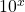,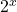, and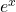. The base number determines how quickly the exponential number grows. The larger the base number the faster the growth.

#### Log10 Plot

Figure 2 shows the linear plot of x versus. In this plot the numberappears exponential on the y-axis for increases in x. The base-10 logarithm returns the exponent of, which is x, which appears linearly on the plot.

Figure 3 is a logarithmic plot on the y-axis, referred to as a semilog plot. The logarithmic plot converts the numbers using log10() before plotting them. Therefore the y-axis shows the exponentialas linear,

(1)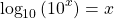and the number x as logarithmic,

(2)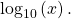#### Log2 Plot

Figure 4 shows the plot for a base-2 exponential. The figure shows a similar shape as Figure 1, just with a base number of 2.

#### Natural Log (Log e) Plot

Figure 4 shows the plot for a base-e exponential. The figure shows a similar shape as Figures 2 and 3, just with a base number of e.

A linear plot will show an exponential number, say, as exponential and a linear number x as linear. However a logarithmic plot, or semilog plot, will show an exponential number as linear and a linear number as logarithmic.